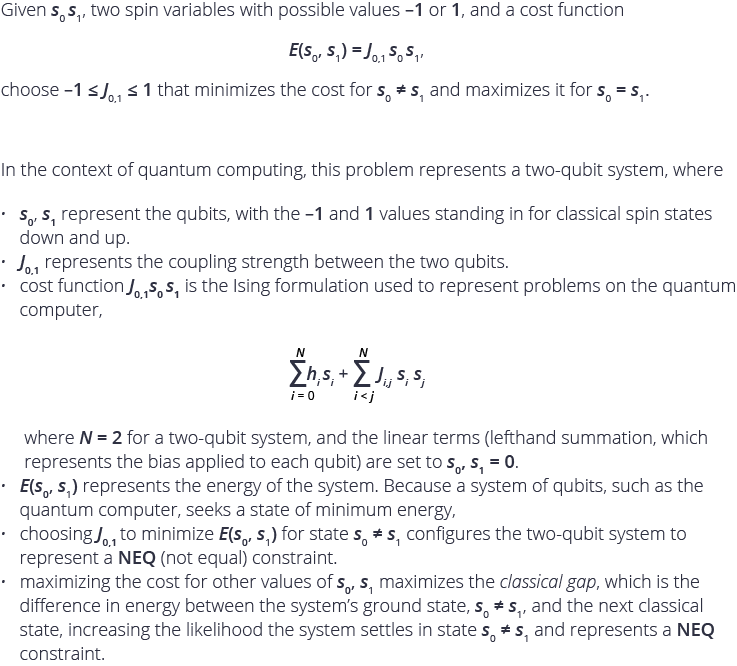# Problem 1: Maximize the Classical Gap of a Two-Qubit SystemHint: See the example of the Boolean NOT gate for solving an analogous problem on a quantum computer.

• J = +1 for anti-ferromagnetic coupling (anti-parallel spins are the lower energy state) and J = -1 for ferromagnetic coupling (parallel spins are the lower energy state).

• Agree to the last answer by @Deepak V. I think J = +1 in this case since the system has minimum energy when the two spin variables are different. The anti-ferromagnetic coupling case.

• Why not just incorporate Maxwell's Demon theory to remove entropy via quantum entanglement. I believe, lasers, magnets, and hanpurple coated qubits could stabilize the qubits and reduce interference to nonexistence, allowing for, commercial quantum Superiority.

• Winner, winner, chicken dinner. Moors law plus/minus 1000 qubits = Quantum Superiority. It important to let the classical computation complete the analysis of the quantum projections in order to save time & relevance. A few resources aside, efforts could be generated towards the economy of scale and the laws of accelerating returns. This would bring quantum computing into exponential acceleration. The real race should be towards open source dapps and quantum hand helds. All of this infrastructure should be built on a blockchain with A.I and edge/fog computing. The data collected will feed into the A.I and manifested via quantum development. Our efforts should be to strive for a resource generating economy built on a reverse game theory society with zero waste. I want a quantum computer to mine crypto and create a decentralized processing center for hashing algorithms in the cryptu sphere and influence those whithin my sphere towards a decentralized economy.

• Is the 2 qubit specification a restriction on the resources to be used? If not, you can make use of embedding methods to maximize your energy gap by allocating s0 and s1 logical qubits to more than one physical qubit.

One example would be to use the K4,4 structure of the chimera so that: 4 qubits are allocated for s0 and 4 for s1.
Specifically: s0 mapped to {0,2,4,6} and s1 to {1,3,5,7} if qubits 0 to 3 are on one side of K4,4 and 4 to 7 on the other.
Couplers between physical qubits allocated for the same logical qubit would use J=-1 and J=+1 otherwise to enforce the NEQ constraint.
In a way, you're using additional qubits for error correction, but effectively increasing the energy gap for the same problem specification.

• Four possible energy states with degeneracy, having energy eigenvalues +J(same spins) & - J(opposite spins). Thus system expresses NEQ constraint if - J is the lowest energy eigenvalue and the next one is + J. So the classical gap is 2J. Hence J = +1 maximizes classical gap.

• I tried coding. I am a new to this. I used the csp approach by adding constraints described D-Wave. I get a 100% probability on s1=1, s2=1 and j=1. Seems like s1 not equal s2 constraint is not working. Can anyone help.

Code is here. Thanks.

• Hello Yasas,

That is an interesting approach to solving this problem! In this case, you might have an easier time calculating the energy of each combination of s1, s2 and J, and looking for the value of J that expresses the NEQ constraint explained in the problem.

• problem #2 is out, so what is the solution for problem #1 and why? :-)

• Thanks to those of you who tried this problem. The answer is 1.

Good answers with good explanations here from several of you, including Deepak, Ruizhe, and Aniruddha.

Please also try Problem 2, posted recently.